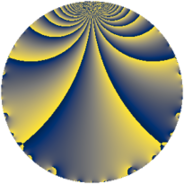# Properties

 Label 5520.2.a.bnLevel $5520$ Weight $2$ Character orbit 5520.a Self dual yes Analytic conductor $44.077$ Analytic rank $1$ Dimension $2$ CM no Inner twists $1$

# Learn more about

## Newspace parameters

 Level: $$N$$ $$=$$ $$5520 = 2^{4} \cdot 3 \cdot 5 \cdot 23$$ Weight: $$k$$ $$=$$ $$2$$ Character orbit: $$[\chi]$$ $$=$$ 5520.a (trivial)

## Newform invariants

 Self dual: yes Analytic conductor: $$44.0774219157$$ Analytic rank: $$1$$ Dimension: $$2$$ Coefficient field: $$\Q(\sqrt{17})$$ Defining polynomial: $$x^{2} - x - 4$$ Coefficient ring: $$\Z[a_1, \ldots, a_{7}]$$ Coefficient ring index: $$1$$ Twist minimal: no (minimal twist has level 2760) Fricke sign: $$1$$ Sato-Tate group: $\mathrm{SU}(2)$

## $q$-expansion

Coefficients of the $$q$$-expansion are expressed in terms of $$\beta = \frac{1}{2}(1 + \sqrt{17})$$. We also show the integral $$q$$-expansion of the trace form.

 $$f(q)$$ $$=$$ $$q + q^{3} - q^{5} + ( 1 + \beta ) q^{7} + q^{9} +O(q^{10})$$ $$q + q^{3} - q^{5} + ( 1 + \beta ) q^{7} + q^{9} -2 q^{11} -2 q^{13} - q^{15} + ( -1 + \beta ) q^{17} + ( -2 - 2 \beta ) q^{19} + ( 1 + \beta ) q^{21} + q^{23} + q^{25} + q^{27} + ( 3 - \beta ) q^{29} + ( -1 - 3 \beta ) q^{31} -2 q^{33} + ( -1 - \beta ) q^{35} + ( -1 - 3 \beta ) q^{37} -2 q^{39} + ( 1 - 3 \beta ) q^{41} - q^{45} + ( 4 - 4 \beta ) q^{47} + ( -2 + 3 \beta ) q^{49} + ( -1 + \beta ) q^{51} + ( -5 - \beta ) q^{53} + 2 q^{55} + ( -2 - 2 \beta ) q^{57} + ( -1 + \beta ) q^{59} + ( -8 + 4 \beta ) q^{61} + ( 1 + \beta ) q^{63} + 2 q^{65} + ( -7 + 3 \beta ) q^{67} + q^{69} + ( -3 + 7 \beta ) q^{71} -10 q^{73} + q^{75} + ( -2 - 2 \beta ) q^{77} + ( -4 + 6 \beta ) q^{79} + q^{81} + ( 1 - 3 \beta ) q^{83} + ( 1 - \beta ) q^{85} + ( 3 - \beta ) q^{87} + 4 \beta q^{89} + ( -2 - 2 \beta ) q^{91} + ( -1 - 3 \beta ) q^{93} + ( 2 + 2 \beta ) q^{95} -14 q^{97} -2 q^{99} +O(q^{100})$$ $$\operatorname{Tr}(f)(q)$$ $$=$$ $$2q + 2q^{3} - 2q^{5} + 3q^{7} + 2q^{9} + O(q^{10})$$ $$2q + 2q^{3} - 2q^{5} + 3q^{7} + 2q^{9} - 4q^{11} - 4q^{13} - 2q^{15} - q^{17} - 6q^{19} + 3q^{21} + 2q^{23} + 2q^{25} + 2q^{27} + 5q^{29} - 5q^{31} - 4q^{33} - 3q^{35} - 5q^{37} - 4q^{39} - q^{41} - 2q^{45} + 4q^{47} - q^{49} - q^{51} - 11q^{53} + 4q^{55} - 6q^{57} - q^{59} - 12q^{61} + 3q^{63} + 4q^{65} - 11q^{67} + 2q^{69} + q^{71} - 20q^{73} + 2q^{75} - 6q^{77} - 2q^{79} + 2q^{81} - q^{83} + q^{85} + 5q^{87} + 4q^{89} - 6q^{91} - 5q^{93} + 6q^{95} - 28q^{97} - 4q^{99} + O(q^{100})$$

## Embeddings

For each embedding $$\iota_m$$ of the coefficient field, the values $$\iota_m(a_n)$$ are shown below.

For more information on an embedded modular form you can click on its label.

Label $$\iota_m(\nu)$$ $$a_{2}$$ $$a_{3}$$ $$a_{4}$$ $$a_{5}$$ $$a_{6}$$ $$a_{7}$$ $$a_{8}$$ $$a_{9}$$ $$a_{10}$$
1.1
 −1.56155 2.56155
0 1.00000 0 −1.00000 0 −0.561553 0 1.00000 0
1.2 0 1.00000 0 −1.00000 0 3.56155 0 1.00000 0
 $$n$$: e.g. 2-40 or 990-1000 Significant digits: Format: Complex embeddings Normalized embeddings Satake parameters Satake angles

## Atkin-Lehner signs

$$p$$ Sign
$$2$$ $$1$$
$$3$$ $$-1$$
$$5$$ $$1$$
$$23$$ $$-1$$

## Inner twists

This newform does not admit any (nontrivial) inner twists.

## Twists

By twisting character orbit
Char Parity Ord Mult Type Twist Min Dim
1.a even 1 1 trivial 5520.2.a.bn 2
4.b odd 2 1 2760.2.a.m 2
12.b even 2 1 8280.2.a.bd 2

By twisted newform orbit
Twist Min Dim Char Parity Ord Mult Type
2760.2.a.m 2 4.b odd 2 1
5520.2.a.bn 2 1.a even 1 1 trivial
8280.2.a.bd 2 12.b even 2 1

## Hecke kernels

This newform subspace can be constructed as the intersection of the kernels of the following linear operators acting on $$S_{2}^{\mathrm{new}}(\Gamma_0(5520))$$:

 $$T_{7}^{2} - 3 T_{7} - 2$$ $$T_{11} + 2$$ $$T_{13} + 2$$ $$T_{17}^{2} + T_{17} - 4$$ $$T_{19}^{2} + 6 T_{19} - 8$$

## Hecke characteristic polynomials

$p$ $F_p(T)$
$2$ $$T^{2}$$
$3$ $$( -1 + T )^{2}$$
$5$ $$( 1 + T )^{2}$$
$7$ $$-2 - 3 T + T^{2}$$
$11$ $$( 2 + T )^{2}$$
$13$ $$( 2 + T )^{2}$$
$17$ $$-4 + T + T^{2}$$
$19$ $$-8 + 6 T + T^{2}$$
$23$ $$( -1 + T )^{2}$$
$29$ $$2 - 5 T + T^{2}$$
$31$ $$-32 + 5 T + T^{2}$$
$37$ $$-32 + 5 T + T^{2}$$
$41$ $$-38 + T + T^{2}$$
$43$ $$T^{2}$$
$47$ $$-64 - 4 T + T^{2}$$
$53$ $$26 + 11 T + T^{2}$$
$59$ $$-4 + T + T^{2}$$
$61$ $$-32 + 12 T + T^{2}$$
$67$ $$-8 + 11 T + T^{2}$$
$71$ $$-208 - T + T^{2}$$
$73$ $$( 10 + T )^{2}$$
$79$ $$-152 + 2 T + T^{2}$$
$83$ $$-38 + T + T^{2}$$
$89$ $$-64 - 4 T + T^{2}$$
$97$ $$( 14 + T )^{2}$$
show more
show less